# 计算统计学与数据科学 07：非线性模型

## 墨尔本大学 MAST90083 课程笔记

Posted by YEY on September 14, 2020

# Lecture 07 非线性模型

• Gareth, J., Daniela, W., Trevor, H., & Robert, T. (2013). An intruduction to statistical learning: with applications in R. Spinger.
• Hastie, T., Tibshirani, R., & Friedman, J. (2009). The elements of statistical learning: data mining, inference, and prediction. Spinger Science & Business Media.

## 1. 引言

• 多项式回归 (Polynomial regression)：用原始预测变量的幂作为新的预测变量以替代原始变量。例如：一个三次回归模型包含三个预测变量 $X,X^2,X^3$。这是一种简单实用的表达数据非线性关系的模型。

• 阶梯函数 (Step functions)：将某个预测变量的取值空间分割成 $K$ 个不同区域，以此来生成一个新的定性变量，分段拟合一个常量函数。

• 回归样条 (Regression spline)：该方法在形式上比多项式回归和阶梯拟合方法更灵活，实际上回归样条可以视为前两类方法的推广。首先将 $X$ 的取值范围分割成 $K$ 个区域，在每个区域上分别独立拟合一个多项式函数。然而，通常会对这些多项式函数进行一些限制以保证在区域边界或 结点 (knots) 处的连接是光滑的。只要将 $X$ 的取值区间划分为足够多的区域，此方法就能够产生灵活度很高的拟合。

• 光滑样条 (Smoothing splines)：与回归样条类似，但是产生机制略有不同，一般是通过最小化一个带光滑惩罚项的残差平方和的式子来得到光滑样条的结果。

• 局部回归 (Local regression)：与样条方法类似，但是存在一个重大差别：局部回归中的区域之间是允许重叠的，并且这种重叠将以一种非常光滑的方式完成。

## 2. 多项式回归

$y_i = \beta_0 + \beta_1 x_i + \beta_2 x_i^2 + \beta_3 x_i^3 + \dots + \beta_d x_i^d + \epsilon_i$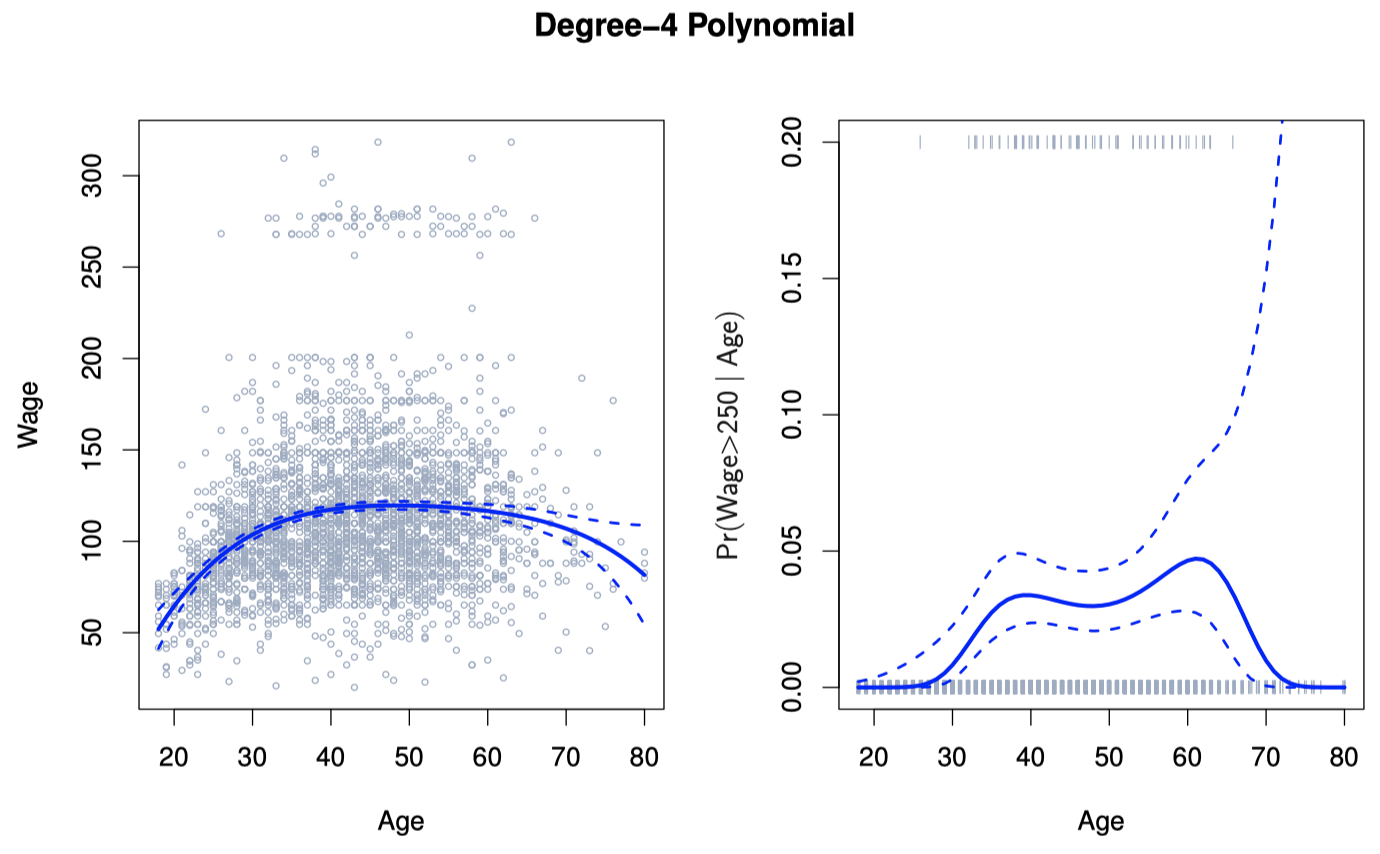$\hat f(x_0) = \hat \beta_0 + \hat \beta_1 x_0+ \hat \beta_2 x_0^2+ \hat \beta_3 x_0^3+ \hat \beta_4 x_0^4$

$\Pr(y_i > 250 \mid x_i) = \dfrac{\exp(\beta_0 + \beta_1 x_i + \beta_2 x_i^2 + \dots + \beta_d x_i^d)}{1 + \exp(\beta_0 + \beta_1 x_i + \beta_2 x_i^2 + \dots + \beta_d x_i^d)}$

## 3. 阶梯函数

\begin{align} C_0(X) &= I(X < c_1) \\[2ex] C_1(X) &= I(c_1 \le X < c_2) \\[2ex] C_2(X) &= I(c_2 \le X < c_3) \\[2ex] &\; \vdots \\[2ex] C_{K-1}(X) &= I(c_{K-1} \le X < c_K) \\[2ex] C_K(X) &= I(c_K \le X) \end{align}

$y_i = \beta_0 + \beta_1 C_1(x_i) + \beta_2 C_2(x_i) + \cdots + \beta_K C_K(x_i) +\epsilon_i$

$\Pr(y_i > 250 \mid x_i) = \dfrac{\exp(\beta_0 + \beta_1 C_1(x_i) + \cdots + \beta_K C_K(x_i))}{1 + \exp(\beta_0 + \beta_1 C_1(x_i) + \cdots + \beta_K C_K(x_i))}$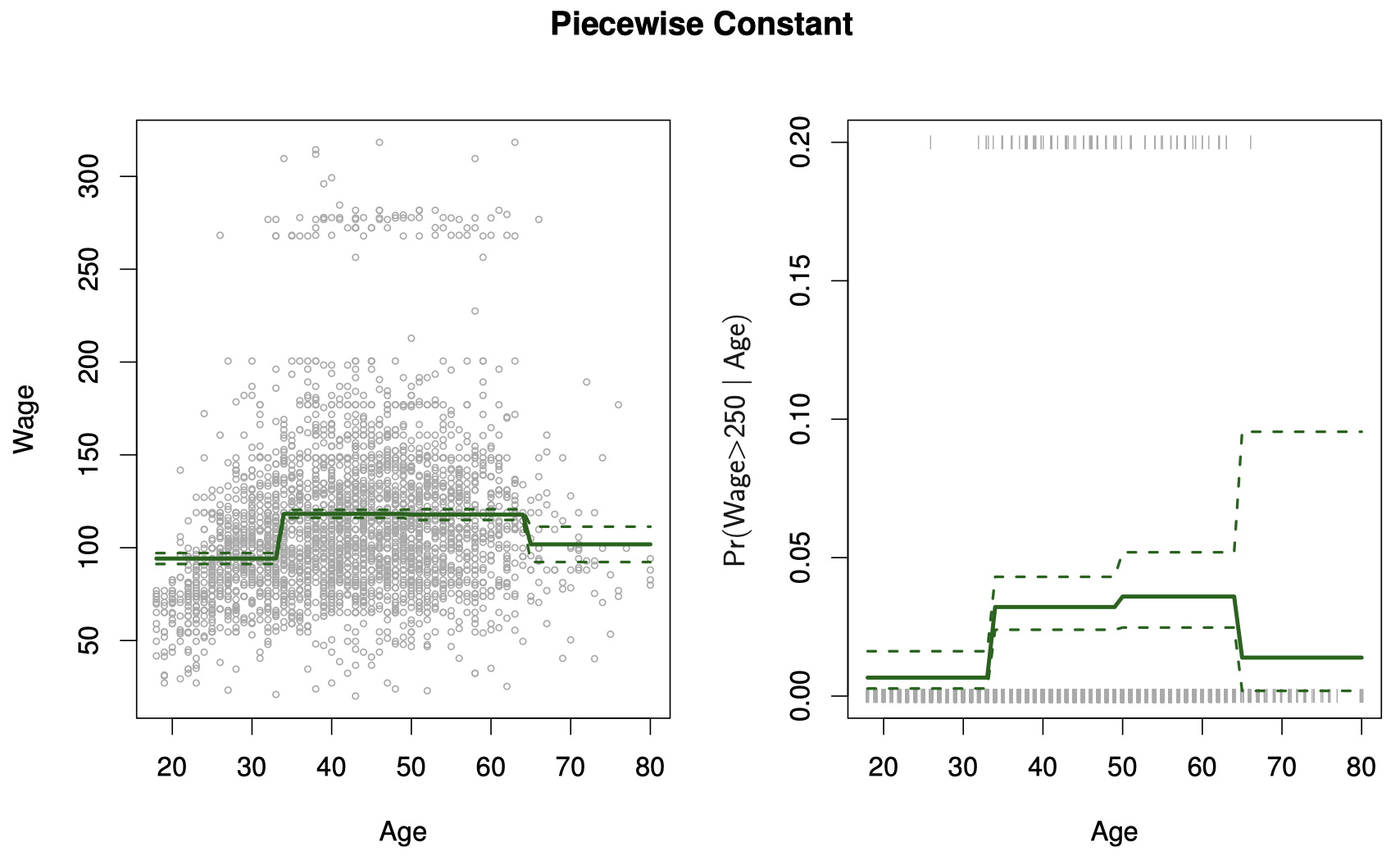$I(\mathtt{year} < 2005)\cdot \mathtt{age},\quad I(\mathtt{year} \ge 2005)\cdot \mathtt{age}$

## 4. 基函数

$y_i = \beta_0 + \beta_1 b_1(x_i) + \beta_2 b_2(x_i) + \beta_3 b_3(x_i) + \cdots + \beta_K b_K(x_i) +\epsilon_i$

## 5. 回归样条

### 5.1 分段多项式

$y_i = \beta_0 + \beta_1 x_i + \beta_2 x_i^2 + \beta_3 x_i^3 + \epsilon_i$

$y_i = \begin{cases}y_i = \beta_{01} + \beta_{11} x_i + \beta_{21} x_i^2 + \beta_{31} x_i^3 + \epsilon_i & \text{if } x_i < c \\[2ex] y_i = \beta_{02} + \beta_{12} x_i + \beta_{22} x_i^2 + \beta_{32} x_i^3 + \epsilon_i & \text{if } x_i \ge c \end{cases}$

### 5.2 约束条件与样条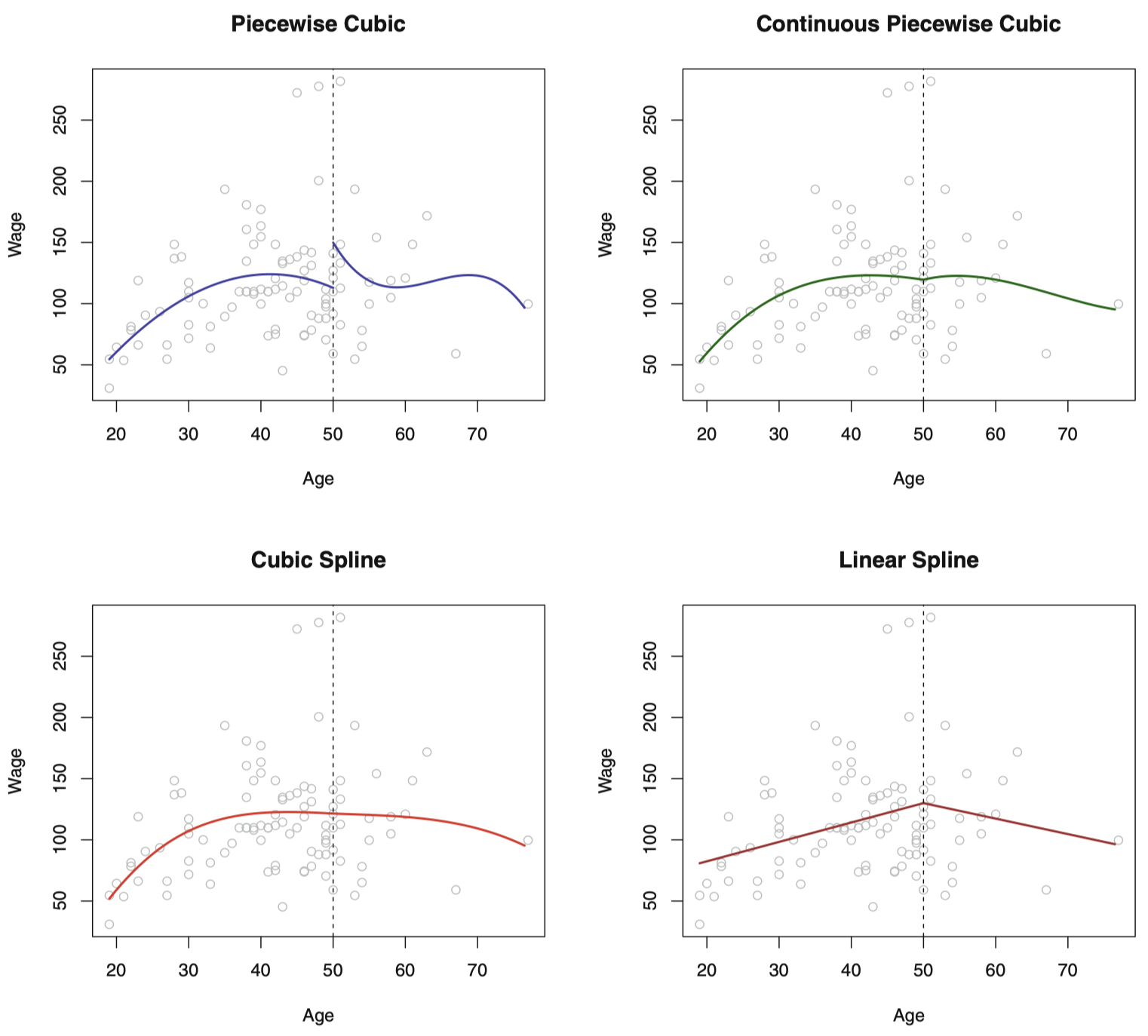### 5.3 样条基函数表示

#### 线性样条

$y_i = \beta_0 + \beta_1 b_1(x_i) + \beta_2 b_2(x_i) + \cdots + \beta_{K+1} b_{K+1}(x_i) + \epsilon_i$

\begin{align} b_1(x_i) &= x_i \\[2ex] b_{k+1}(x_i) &= (x_i - \xi_k)_{+} \quad k=1,\dots,K \end{align}

$(x_i - \xi_k)_{+} = \begin{cases}x_i - \xi_k & \text{if } x_i > \xi_k \\[2ex] 0 & \text{otherwise}\end{cases}$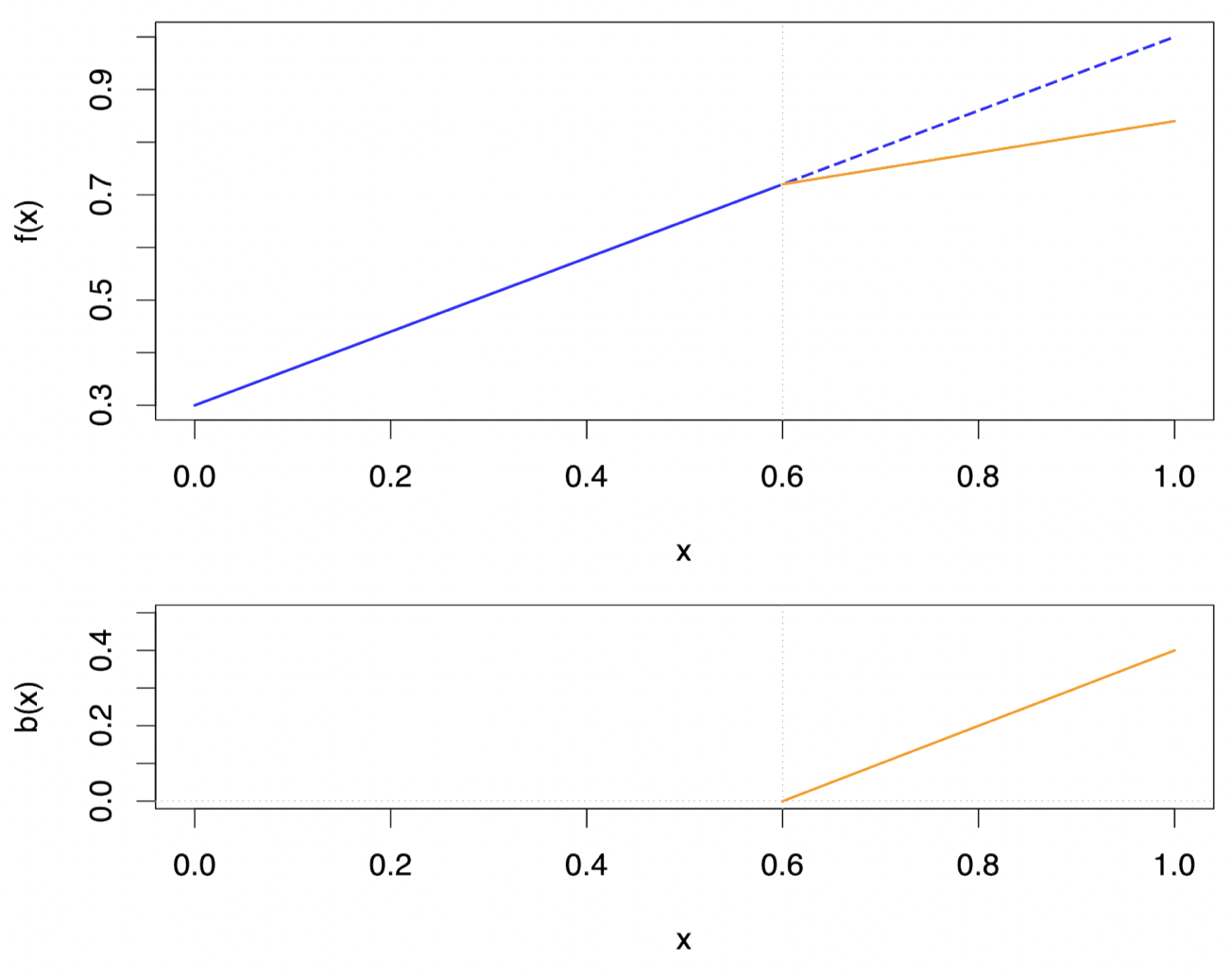#### 三次样条

$y_i = \beta_0 + \beta_1 b_1(x_i) + \beta_2 b_2(x_i) + \cdots + \beta_{K+3} b_{K+3}(x_i) + \epsilon_i$

\begin{align} b_1(x_i) &= x_i \\[2ex] b_2(x_i) &= x_i^2 \\[2ex] b_3(x_i) &= x_i^3 \\[2ex] b_{k+3}(x_i) &= (x_i - \xi_k)_{+}^3 \quad k=1,\dots,K \end{align}

$(x_i-\xi_k)_{+}^3 = \begin{cases}(x_i-\xi_k)^3 & \text{if } x_i > \xi_k \\[2ex] 0 & \text{otherwise}\end{cases}$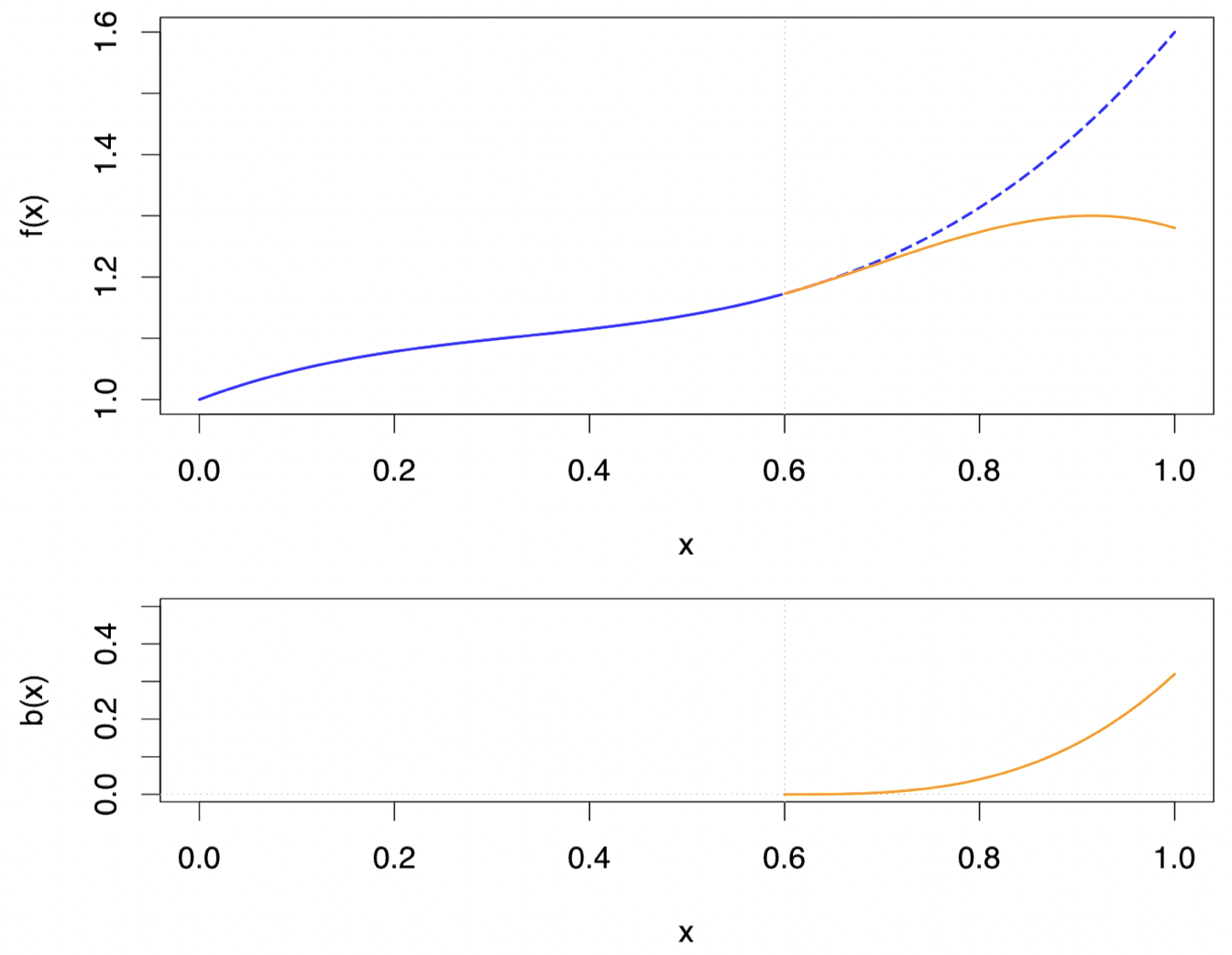#### 自然样条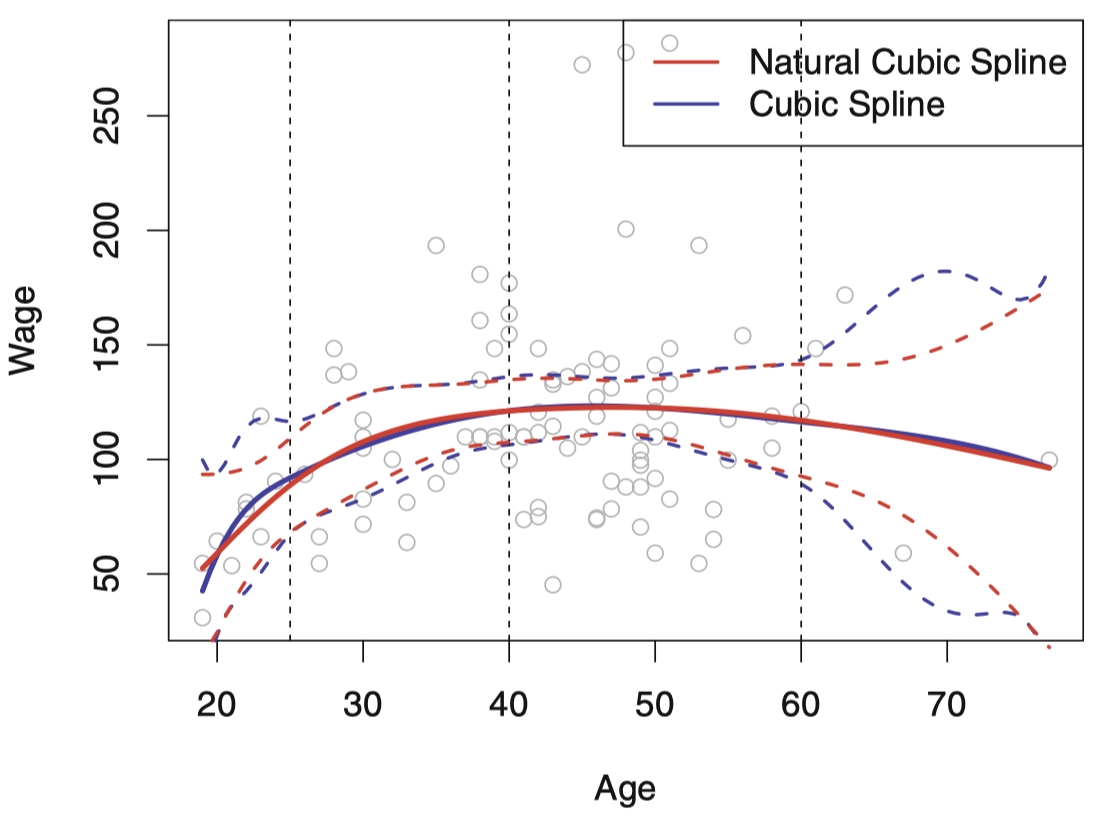### 5.4 确定结点的个数和位置

• 一种方式是先指定所需的结点数 $K$，然后将它们放置在观测 $X$ 的合适的分位数上。
• 一个包含 $K$ 个结点的三次样条具有 $K+4$ 个参数或者自由度。
• 一个包含 $K$ 个结点的自然样条具有 $K$ 个自由度。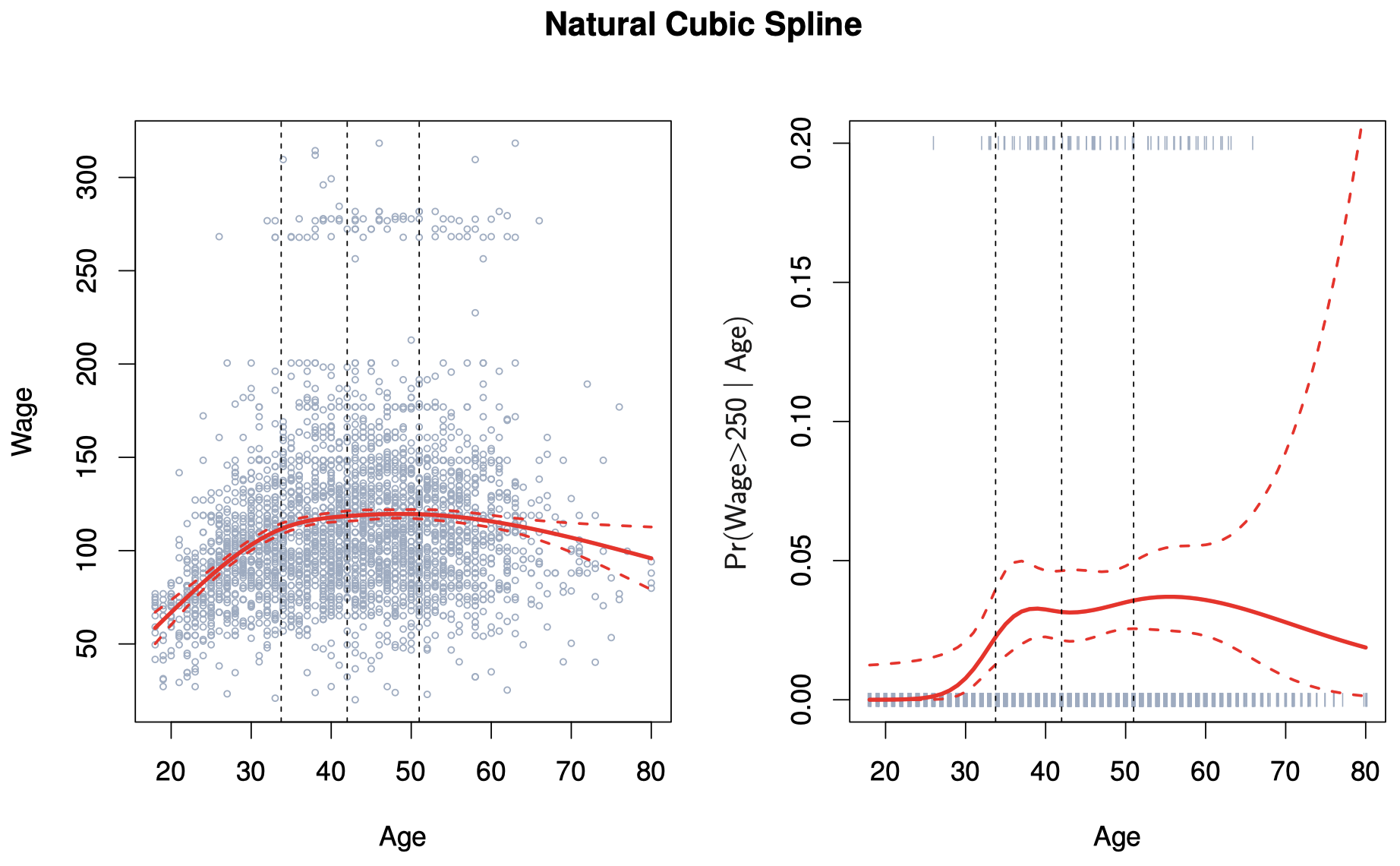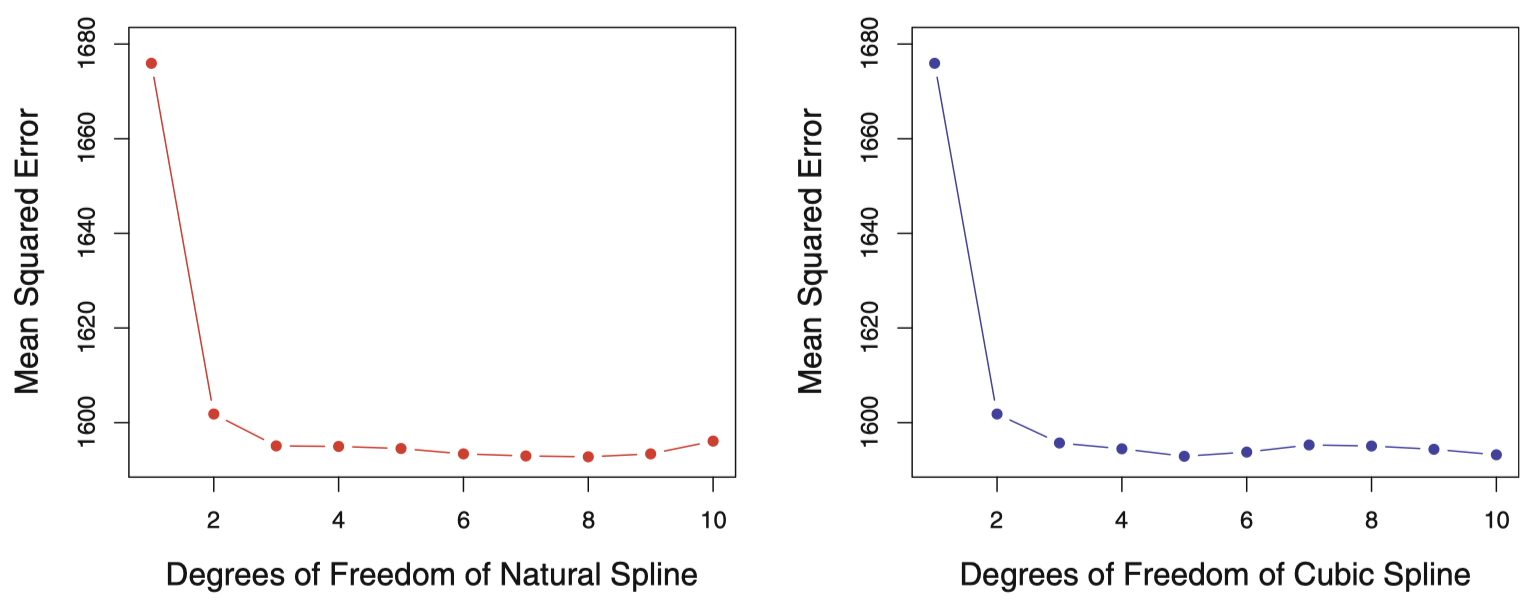### 5.5 对比多项式回归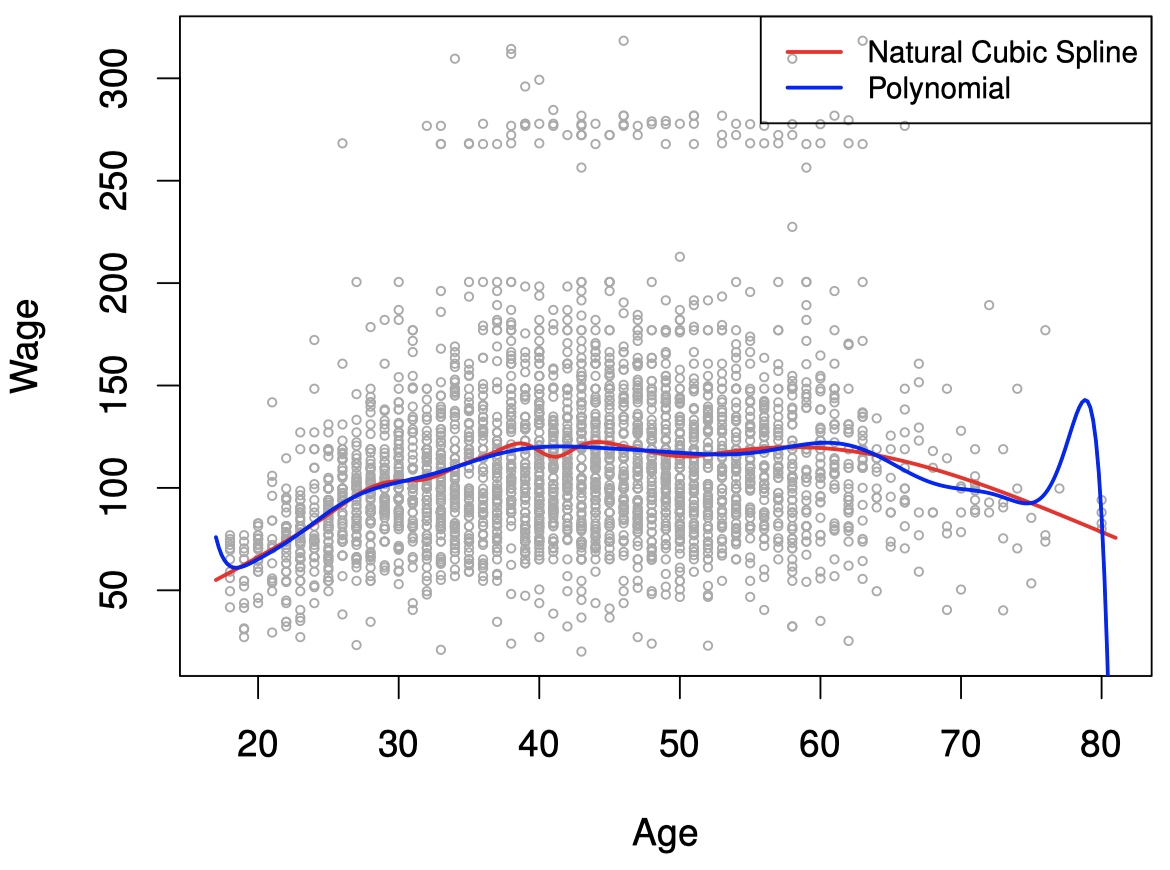## 6. 光滑样条

### 6.1 光滑样条概述

$\mathop{\operatorname{minimize}}\limits_{g \in \mathcal S} \sum_{i=1}^{n}(y_i - g(x_i))^2 + \lambda \int g''(t)^2dt$

• 第一项是 $\mathrm{RSS}$，它试图使 $g(x)$ 在每个 $x_i$ 上匹配数据。
• 第二项是 粗糙度惩罚 (roughness penalty)，它控制着 $g(x)$ 的波动程度，通过一个 调节参数 $\lambda \ge 0$ 进行调节：
• $\lambda$ 越小，函数波动越剧烈，当 $\lambda=0$ 时，将最终得到在 $y_i$ 处插值的函数。
• 随着 $\lambda \to \infty$，函数 $g(x)$ 趋近线性。

### 6.2 选择光滑参数 $\lambda$

$\hat{\mathbf g}_{\lambda} =\mathbf S_{\lambda}\mathbf y$

$\mathrm{df}_{\lambda}=\sum_{i=1}^{n}\{\mathbf{S}_{\lambda}\}_{ii}$

$\mathrm{RSS}_{cv}(\lambda) = \sum_{i=1}^{n}(y_i - \hat g_{\lambda}^{(-i)}(x_i))^2 = \sum_{i=1}^{n}\left[\dfrac{y_i - \hat g_{\lambda}(x_i)}{1-\{\mathbf S_{\lambda}\}_{ii}} \right]^2$

• 光滑样条避免了结点选择问题，只需要选择一个 $\lambda$ 即可。
• 具体算法细节过于复杂，此处略去。在 R 中，可以用 smooth.spline() 来拟合光滑样条。
• 在 R 中，我们可以直接指定自由度来拟合光滑样条 smooth.spline(age, wage, df=10)
• 在 R 中，默认采用 LOOCV 来拟合光滑样条 smooth.spline(age, wage)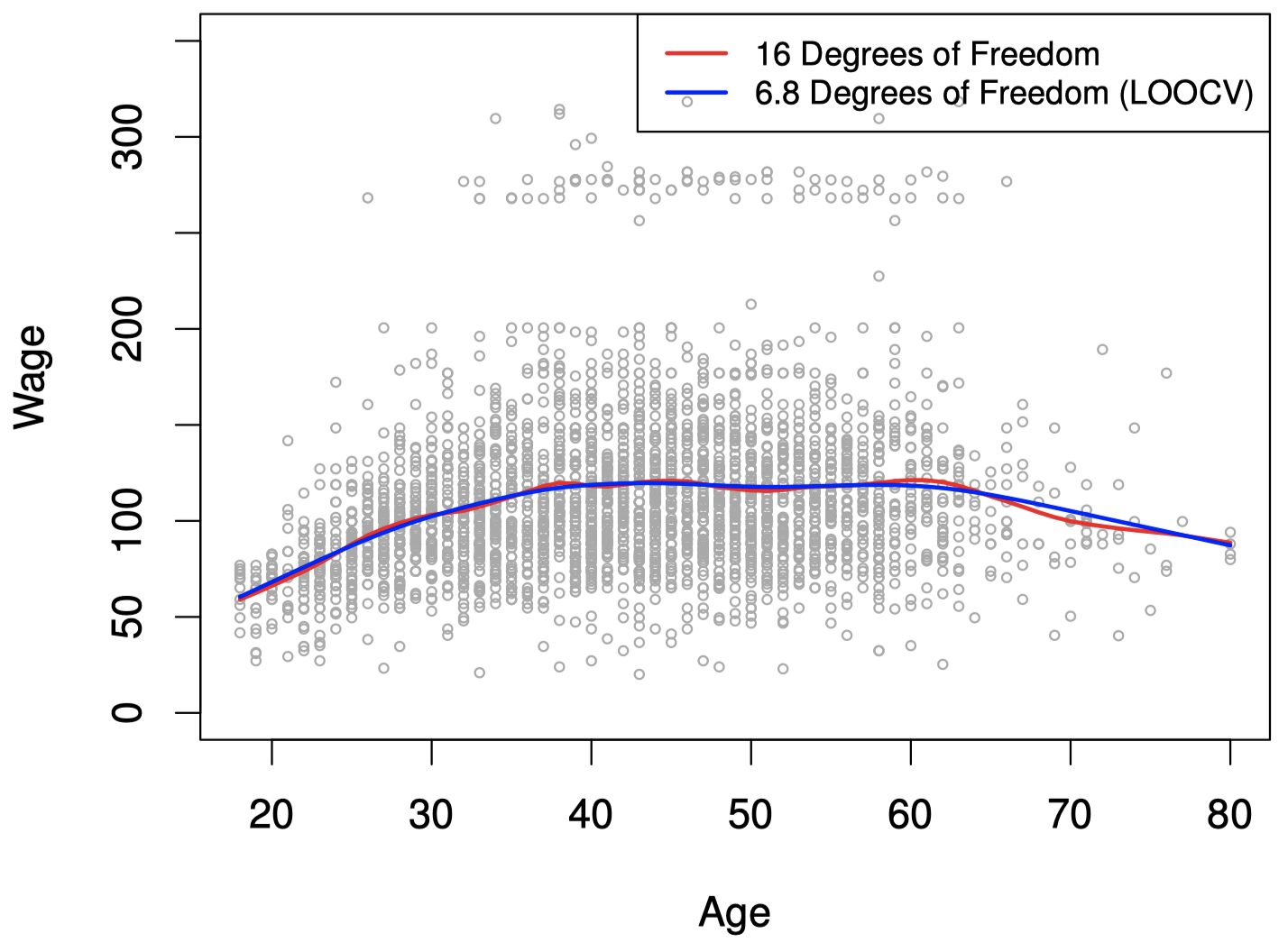## 7. 局部回归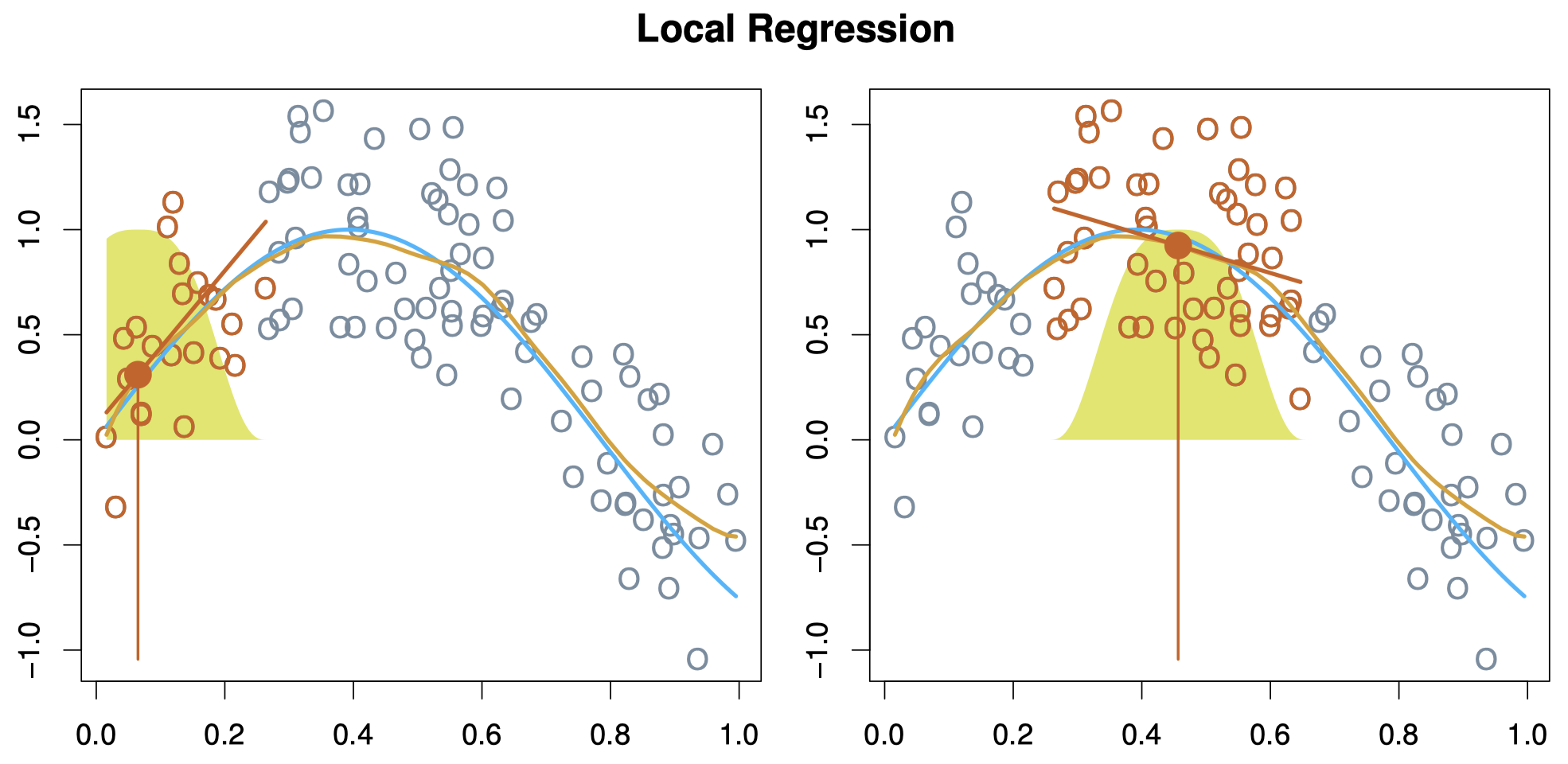## 8. 广义可加模型

$y_i=\beta_0 + f_1(x_{i1})+ f_2(x_{i2})+\cdots + f_p(x_{ip}) + \epsilon_i$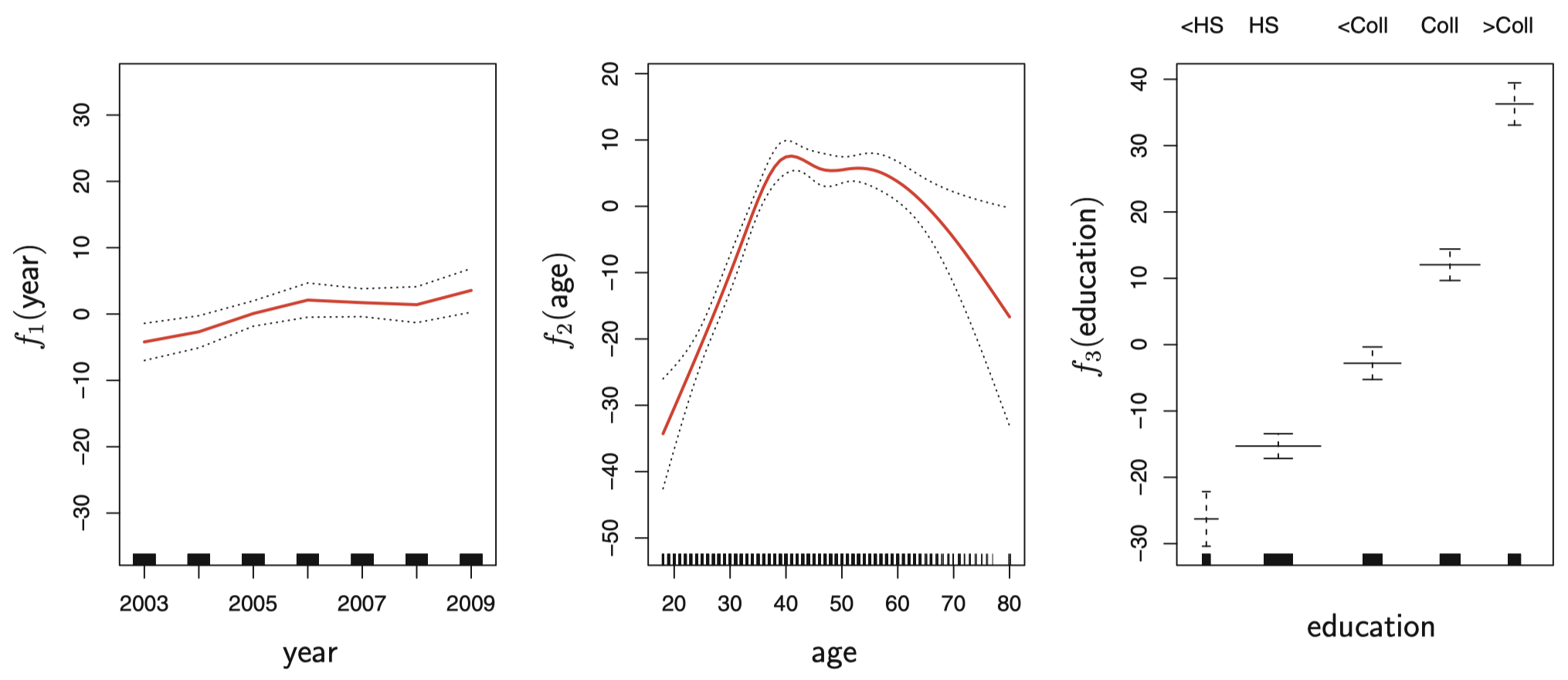GAM 用于分类：

$\log \left(\dfrac{p(X)}{1-p(X)} \right) = \beta_0 + f_1(X_1)+ f_2(X_2)+\cdots + f_p(X_p)$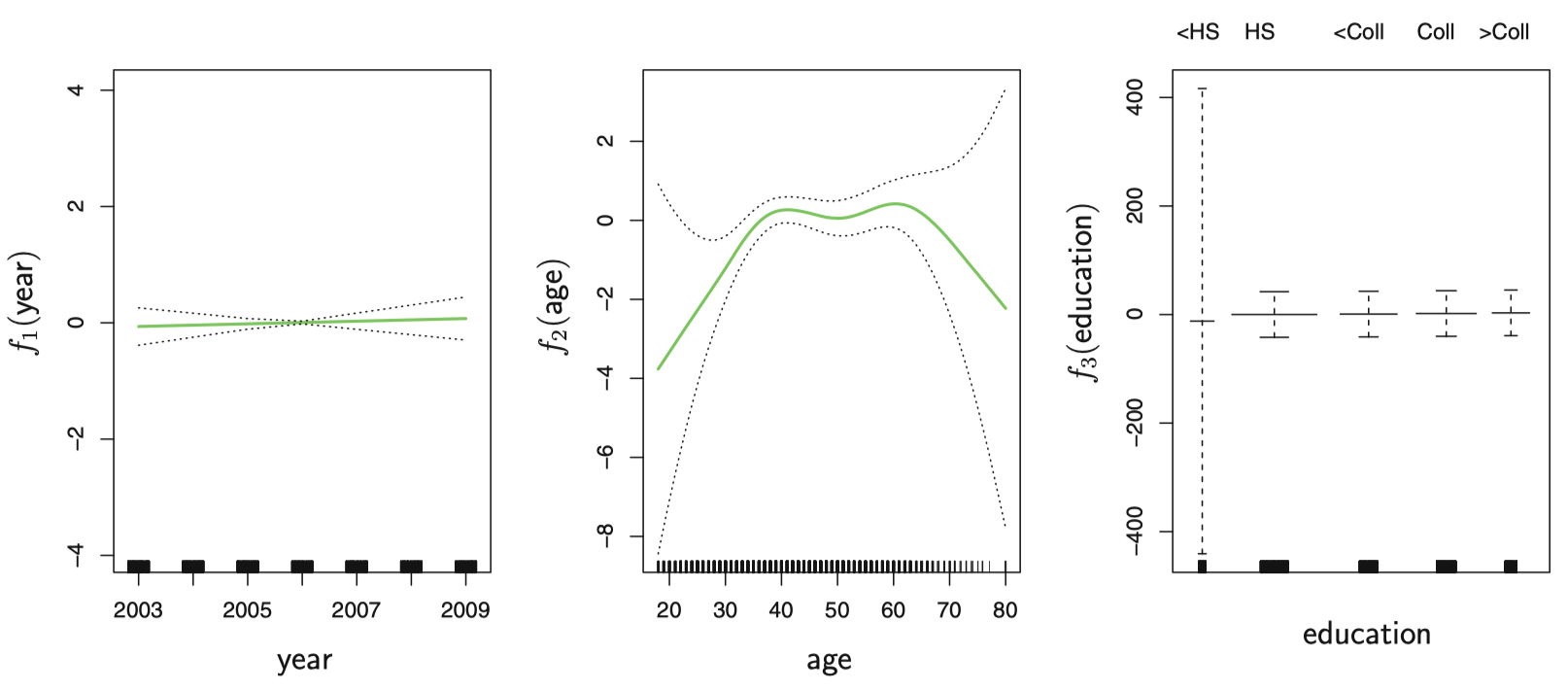本作品采用知识共享署名-非商业性使用-相同方式共享 4.0 国际许可协议进行许可。 欢迎转载，并请注明来自：YEY 的博客 同时保持文章内容的完整和以上声明信息！